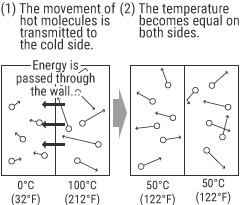# What Is Temperature?

Temperature is an indicator that shows the state of a substance such as hot and cold. Temperature is expressed in units such as Kelvin (K), Celsius (°C), and Fahrenheit (°F).
This section explains the mechanism where heat, which is closely related to temperature, is generated and the units of measurement.

## Difference between temperature and heatFirst, let’s figure out what temperature and heat are.
All substances (molecules) have energy generated by vibration or other factors. “Heat” is a form of energy.
Then, what is the difference between “temperature” and “heat”?
“Temperature” is a scale to measure “heat” within a substance—namely, a quantification of the state of the heat energy.
Because heat energy moves between substances having different temperatures, a hot substance and a cold substance come to the same temperature after being kept in contact with each other.

### Units of temperature

There are various units of temperature, including degrees Celsius (°C), degrees Fahrenheit (°F), and Kelvin (K, absolute temperature, which is mainly used in thermodynamics). These three units of temperature are compared as follows.

Kelvin Degrees Celsius Degrees Fahrenheit
Absolute zero
0K
-273.15°C
-459.67°F
Fahrenheit’s ice/salt mixture
255.37K
-17.78°C
0°F
Ice melts (in standard state)
273.15K
0°C
32°F
Water boils (in standard state)
373.15K
100°C
212°F

INDEX